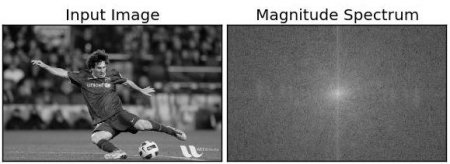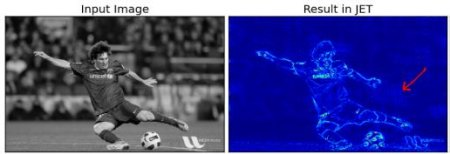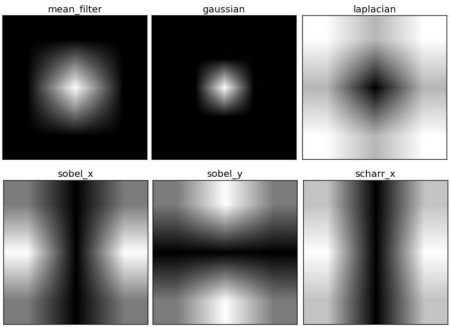# 傅里叶变换

### Numpy中的傅里叶变换

import cv2 as cv
import numpy as np
from matplotlib import pyplot as plt
f = np.fft.fft2(img)
fshift = np.fft.fftshift(f)
magnitude_spectrum = 20*np.log(np.abs(fshift))
plt.subplot(121),plt.imshow(img, cmap = 'gray')
plt.title('Input Image'), plt.xticks([]), plt.yticks([])
plt.subplot(122),plt.imshow(magnitude_spectrum, cmap = 'gray')
plt.title('Magnitude Spectrum'), plt.xticks([]), plt.yticks([])
plt.show()
Result look like below:rows, cols = img.shape
crow,ccol = rows//2 , cols//2
fshift[crow-30:crow+31, ccol-30:ccol+31] = 0
f_ishift = np.fft.ifftshift(fshift)
img_back = np.fft.ifft2(f_ishift)
img_back = np.real(img_back)
plt.subplot(131),plt.imshow(img, cmap = 'gray')
plt.title('Input Image'), plt.xticks([]), plt.yticks([])
plt.subplot(132),plt.imshow(img_back, cmap = 'gray')
plt.title('Image after HPF'), plt.xticks([]), plt.yticks([])
plt.subplot(133),plt.imshow(img_back)
plt.title('Result in JET'), plt.xticks([]), plt.yticks([])
plt.show()### OpenCV中的傅里叶变换

OpenCV为此提供了cv.dft()和cv.idft()函数。它返回与前一个相同的结果，但是有两个通道。第一个通道是结果的实部，第二个通道是结果的虚部。输入图像首先应转换为np.float32。我们来看看怎么做。

import numpy as np
import cv2 as cv
from matplotlib import pyplot as plt
dft = cv.dft(np.float32(img),flags = cv.DFT_COMPLEX_OUTPUT)
dft_shift = np.fft.fftshift(dft)
magnitude_spectrum = 20*np.log(cv.magnitude(dft_shift[:,:,0],dft_shift[:,:,1]))
plt.subplot(121),plt.imshow(img, cmap = 'gray')
plt.title('Input Image'), plt.xticks([]), plt.yticks([])
plt.subplot(122),plt.imshow(magnitude_spectrum, cmap = 'gray')
plt.title('Magnitude Spectrum'), plt.xticks([]), plt.yticks([])
plt.show()


rows, cols = img.shape
crow,ccol = rows/2 , cols/2
# 首先创建一个掩码，中心正方形为1，其余全为零
# 应用掩码和逆DFT
f_ishift = np.fft.ifftshift(fshift)
img_back = cv.idft(f_ishift)
img_back = cv.magnitude(img_back[:,:,0],img_back[:,:,1])
plt.subplot(121),plt.imshow(img, cmap = 'gray')
plt.title('Input Image'), plt.xticks([]), plt.yticks([])
plt.subplot(122),plt.imshow(img_back, cmap = 'gray')
plt.title('Magnitude Spectrum'), plt.xticks([]), plt.yticks([])
plt.show()


### DFT的性能优化

In : img = cv.imread('messi5.jpg',0)
In : rows,cols = img.shape
In : print("{} {}".format(rows,cols))
342 548
In : nrows = cv.getOptimalDFTSize(rows)
In : ncols = cv.getOptimalDFTSize(cols)
In : print("{} {}".format(nrows,ncols))
360 576


nimg = np.zeros((nrows,ncols))
nimg[:rows,:cols] = img


right = ncols - cols
bottom = nrows - rows
bordertype = cv.BORDER_CONSTANT ＃只是为了避免PDF文件中的行中断
nimg = cv.copyMakeBorder(img,0,bottom,0,right,bordertype, value = 0)


In : %timeit fft1 = np.fft.fft2(img)
10 loops, best of 3: 40.9 ms per loop
In : %timeit fft2 = np.fft.fft2(img,[nrows,ncols])
100 loops, best of 3: 10.4 ms per loop


In : %timeit dft1= cv.dft(np.float32(img),flags=cv.DFT_COMPLEX_OUTPUT)
100 loops, best of 3: 13.5 ms per loop
In : %timeit dft2= cv.dft(np.float32(nimg),flags=cv.DFT_COMPLEX_OUTPUT)
100 loops, best of 3: 3.11 ms per loop


### 为什么拉普拉斯算子是高通滤波器？

import cv2 as cv
import numpy as np
from matplotlib import pyplot as plt
# 没有缩放参数的简单均值滤波器
mean_filter = np.ones((3,3))
# 创建高斯滤波器
x = cv.getGaussianKernel(5,10)
gaussian = x*x.T
# 不同的边缘检测滤波器
# x方向上的scharr
scharr = np.array([[-3, 0, 3],
[-10,0,10],
[-3, 0, 3]])
# x方向上的sobel
sobel_x= np.array([[-1, 0, 1],
[-2, 0, 2],
[-1, 0, 1]])
# y方向上的sobel
sobel_y= np.array([[-1,-2,-1],
[0, 0, 0],
[1, 2, 1]])
# 拉普拉斯变换
laplacian=np.array([[0, 1, 0],
[1,-4, 1],
[0, 1, 0]])
filters = [mean_filter, gaussian, laplacian, sobel_x, sobel_y, scharr]
filter_name = ['mean_filter', 'gaussian','laplacian', 'sobel_x', \
'sobel_y', 'scharr_x']
fft_filters = [np.fft.fft2(x) for x in filters]
fft_shift = [np.fft.fftshift(y) for y in fft_filters]
mag_spectrum = [np.log(np.abs(z)+1) for z in fft_shift]
for i in xrange(6):
plt.subplot(2,3,i+1),plt.imshow(mag_spectrum[i],cmap = 'gray')
plt.title(filter_name[i]), plt.xticks([]), plt.yticks([])
plt.show()### 附加资源

1.傅里叶变换的直观解释：http://cns-alumni.bu.edu/~slehar/fourier/fourier.html by Steven Lehar 2. 傅里叶变换：http://homepages.inf.ed.ac.uk/rbf/HIPR2/fourier.htm at HIPR 3. 图像中的频率域指什么？http://dsp.stackexchange.com/q/1637/818